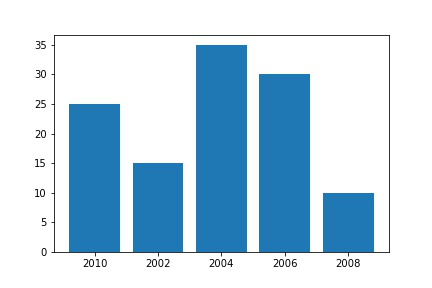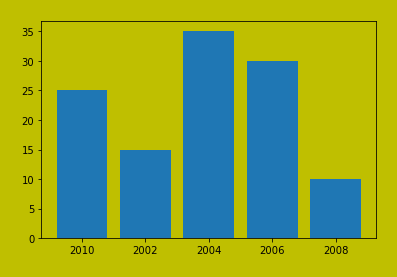Open In App

# How to Save a Plot to a File Using Matplotlib?

Matplotlib is a widely used Python library to plot graphs, plots, charts, etc. show() method is used to display graphs as output, but don’t save it in any file.

## Method 1: Save Plot as Image with Matplotlib using savefig()

The figure produced after data plotting is saved using the savefig() method, as the name implies. Using this technique, the generated figure can be saved to our local computers. the syntax of savefig() is given below:

### Syntax of savefig() function

Syntax: pyplot.savefig(fname, dpi=None, facecolor=’w’, edgecolor=’w’, orientation=’portrait’, papertype=None, format=None, transparent=False, bbox_inches=None, pad_inches=0.1, frameon=None, metadata=None)

Parameters:

• fname : path or name of output file with extension. If extension is not provided plot is saved as png file. Supported file formats: eps, jpeg, jpg, pdf, pgf, png, ps, raw, rgba, svg, svgz, tif, tiff.
• dpi : dots per inch resolution of figure
• facecolor : facecolor of figure
• edgecolor :  edgecolor of figure
• orientation  : landscape or portrait
• format : The file format, e.g. ‘png’, ‘pdf’, ‘svg’, etc.
• transparent : If  it is True, the patches of axes will all be transparent

Return: Save an array as an image file.

In this example, we are creating our own data list, and using Matplotlib we are plotting a bar graph and saving it to the same directory. To save generated graphs in a file on a storage disk, savefig() method is used.

## Python3

 `import` `matplotlib.pyplot as plt` `# Creating data``year ``=` `[``'2010'``, ``'2002'``, ``'2004'``, ``'2006'``, ``'2008'``]``production ``=` `[``25``, ``15``, ``35``, ``30``, ``10``]` `# Plotting barchart``plt.bar(year, production)` `# Saving the figure.``plt.savefig(``"output.jpg"``)` `# Saving figure by changing parameter values``plt.savefig(``"output1"``, facecolor``=``'y'``, bbox_inches``=``"tight"``,``            ``pad_inches``=``0.3``, transparent``=``True``)`

Output:## Method 2: Save Plot as Image with Matplotlib using matplotlib.pyplot.imsave()

Using the matplotlib.pyplot.imsave() method, we may save the plot to an image file rather than using Matplotlib to display it. The arrays are saved using this manner as picture files.

### Syntax of imsave()

Syntax: matplotlib.pyplot.imsave(fname, arr, **kwargs

Parameter:

• fname: A path or a file-like object to store the image.
• arr: The image data.

Return: Save an array as an image file.

In this method, we are trying to read an image using imread() function, and save the same image with a different name using imsave().

## Python3

 `import` `imageio``import` `matplotlib.pyplot as plt``image ``=` `imageio.imread(``'img.png'``)``plt.imshow(image)``plt.imsave(``'img_new.jpg'``,image )` `image_new ``=` `imageio.imread(``'img_new.jpg'``)``plt.imshow(image_new)`

Output:img_new